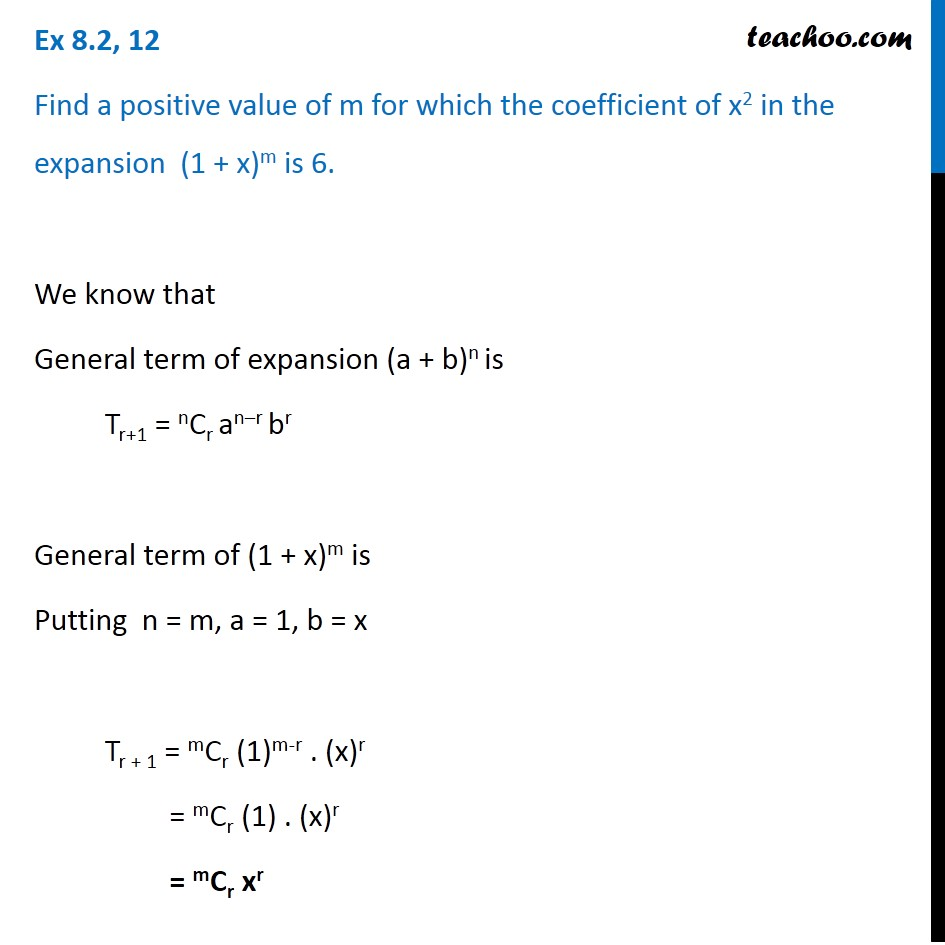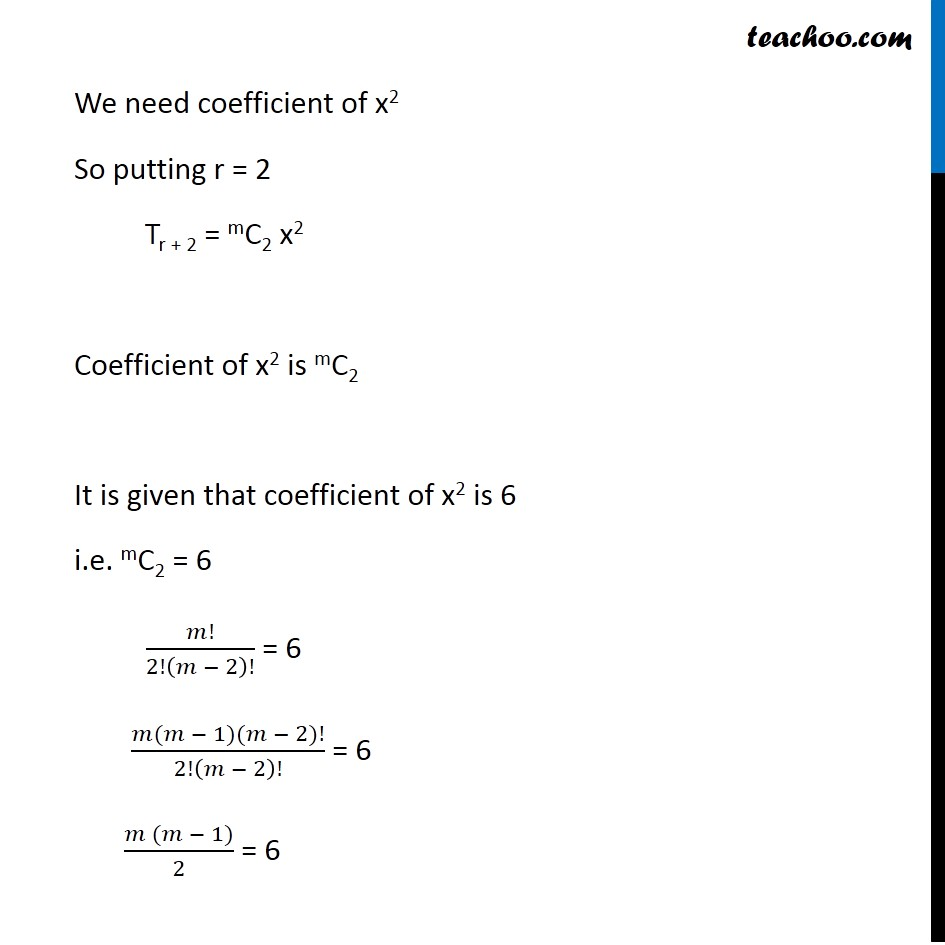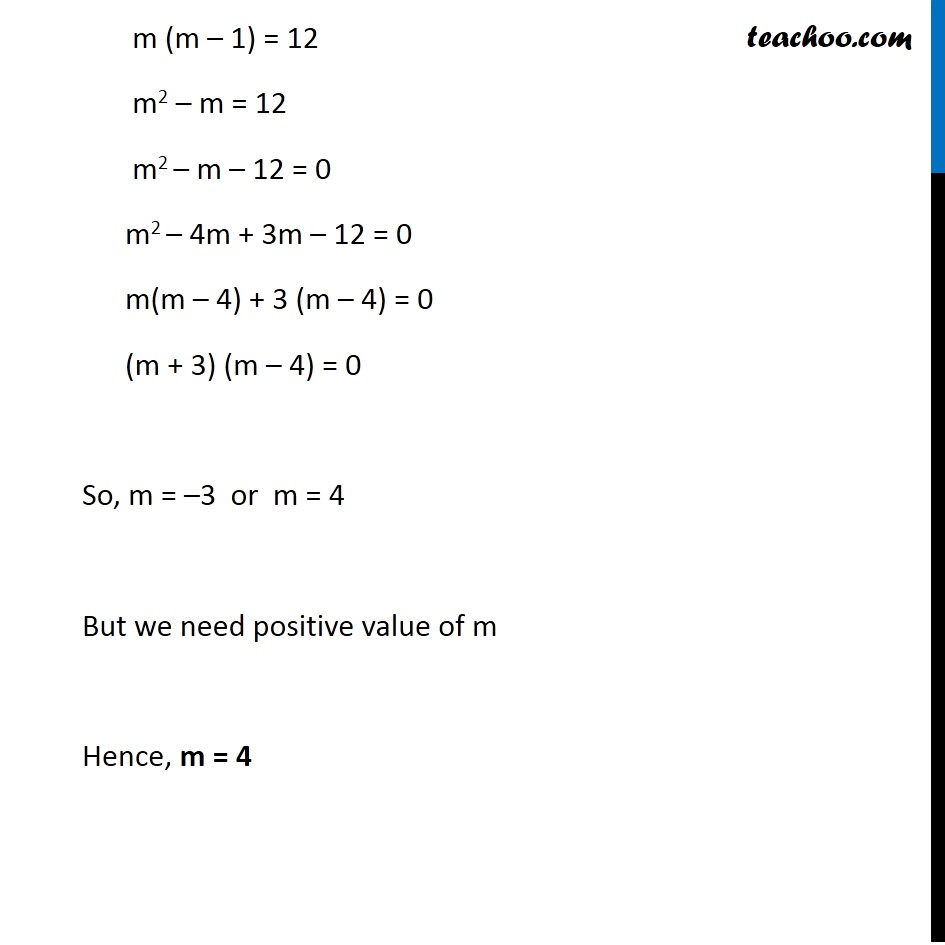General Term of Binomial Theorem

Chapter 7 Class 11 Binomial Theorem
Serial order wiseLearn in your speed, with individual attention - Teachoo Maths 1-on-1 Class

### Transcript

Question 12 Find a positive value of m for which the coefficient of x2 in the expansion (1 + x)m is 6. We know that General term of expansion (a + b)n is Tr+1 = nCr an–r br General term of (1 + x)m is Putting n = m, a = 1, b = x Tr + 1 = mCr (1)m-r . (x)r = mCr (1) . (x)r = mCr xr We need coefficient of x2 So putting r = 2 Tr + 2 = mC2 x2 Coefficient of x2 is mC2 It is given that coefficient of x2 is 6 i.e. mC2 = 6 𝑚!/2!(𝑚 − 2)! = 6 (𝑚(𝑚 − 1)(𝑚 − 2)!)/2!(𝑚 − 2)! = 6 (𝑚 (𝑚 − 1))/2 = 6 m (m – 1) = 12 m2 – m = 12 m2 – m – 12 = 0 m2 – 4m + 3m – 12 = 0 m(m – 4) + 3 (m – 4) = 0 (m + 3) (m – 4) = 0 So, m = –3 or m = 4 But we need positive value of m Hence, m = 4